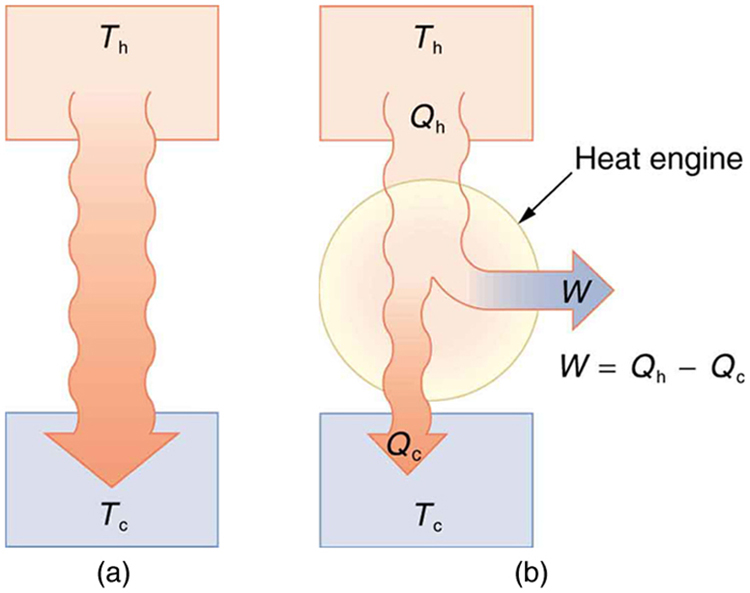# 15.3 Introduction to the second law of thermodynamics: heat engines  (Page 2/10)

 Page 2 / 10

The already familiar direction of heat transfer from hot to cold is the basis of our first version of the second law of thermodynamics    .

## The second law of thermodynamics (first expression)

Heat transfer occurs spontaneously from higher- to lower-temperature bodies but never spontaneously in the reverse direction.

Another way of stating this: It is impossible for any process to have as its sole result heat transfer from a cooler to a hotter object.

## Heat engines

Now let us consider a device that uses heat transfer to do work. As noted in the previous section, such a device is called a heat engine, and one is shown schematically in [link] (b). Gasoline and diesel engines, jet engines, and steam turbines are all heat engines that do work by using part of the heat transfer from some source. Heat transfer from the hot object (or hot reservoir) is denoted as ${Q}_{\text{h}}$ , while heat transfer into the cold object (or cold reservoir) is ${Q}_{\text{c}}$ , and the work done by the engine is $W$ . The temperatures of the hot and cold reservoirs are ${T}_{\text{h}}$ and ${T}_{\text{c}}$ , respectively.(a) Heat transfer occurs spontaneously from a hot object to a cold one, consistent with the second law of thermodynamics. (b) A heat engine, represented here by a circle, uses part of the heat transfer to do work. The hot and cold objects are called the hot and cold reservoirs. Q h size 12{Q rSub { size 8{h} } } {} is the heat transfer out of the hot reservoir, W size 12{W} {} is the work output, and Q c size 12{Q rSub { size 8{c} } } {} is the heat transfer into the cold reservoir.

Because the hot reservoir is heated externally, which is energy intensive, it is important that the work is done as efficiently as possible. In fact, we would like $W$ to equal ${Q}_{\text{h}}$ , and for there to be no heat transfer to the environment ( ${Q}_{\text{c}}=0$ ). Unfortunately, this is impossible. The second law of thermodynamics    also states, with regard to using heat transfer to do work (the second expression of the second law):

## The second law of thermodynamics (second expression)

It is impossible in any system for heat transfer from a reservoir to completely convert to work in a cyclical process in which the system returns to its initial state.

A cyclical process    brings a system, such as the gas in a cylinder, back to its original state at the end of every cycle. Most heat engines, such as reciprocating piston engines and rotating turbines, use cyclical processes. The second law, just stated in its second form, clearly states that such engines cannot have perfect conversion of heat transfer into work done. Before going into the underlying reasons for the limits on converting heat transfer into work, we need to explore the relationships among $W$ , ${Q}_{\text{h}}$ , and ${Q}_{\text{c}}$ , and to define the efficiency of a cyclical heat engine. As noted, a cyclical process brings the system back to its original condition at the end of every cycle. Such a system’s internal energy $U$ is the same at the beginning and end of every cycle—that is, $\Delta U=0$ . The first law of thermodynamics states that

$\Delta U=Q-W\text{,}$

where $Q$ is the net heat transfer during the cycle ( $Q={Q}_{\text{h}}-{Q}_{\text{c}}$ ) and $W$ is the net work done by the system. Since $\Delta U=0$ for a complete cycle, we have

full meaning of GPS system
how to prove that Newton's law of universal gravitation F = GmM ______ R²
sir dose it apply to the human system
prove that the centrimental force Fc= M1V² _________ r
prove that centripetal force Fc = MV² ______ r
Kaka
how lesers can transmit information
griffts bridge derivative
below me
please explain; when a glass rod is rubbed with silk, it becomes positive and the silk becomes negative- yet both attracts dust. does dust have third types of charge that is attracted to both positive and negative
what is a conductor
Timothy
hello
Timothy
below me
why below you
Timothy
no....I said below me ...... nothing below .....ok?
dust particles contains both positive and negative charge particles
Mbutene
corona charge can verify
Stephen
when pressure increases the temperature remain what?
remains the temperature
betuel
what is frequency
define precision briefly
CT scanners do not detect details smaller than about 0.5 mm. Is this limitation due to the wavelength of x rays? Explain.
hope this helps
what's critical angle
The Critical Angle Derivation So the critical angle is defined as the angle of incidence that provides an angle of refraction of 90-degrees. Make particular note that the critical angle is an angle of incidence value. For the water-air boundary, the critical angle is 48.6-degrees.
okay whatever
Chidalu
pls who can give the definition of relative density?
Temiloluwa
the ratio of the density of a substance to the density of a standard, usually water for a liquid or solid, and air for a gas.
Chidalu
What is momentum
mass ×velocity
Chidalu
it is the product of mass ×velocity of an object
Chidalu
how do I highlight a sentence]p? I select the sentence but get options like copy or web search but no highlight. tks. src
then you can edit your work anyway you want
Wat is the relationship between Instataneous velocity
Instantaneous velocity is defined as the rate of change of position for a time interval which is almost equal to zero
AstronomyByBy Madison ChristianBy Brooke DelaneyBy OpenStaxBy Marriyam RanaBy Marion CabalfinBy Mistry BhaveshBy OpenStaxBy Jessica CollettBy Richley CrapoBy Stephen Voron